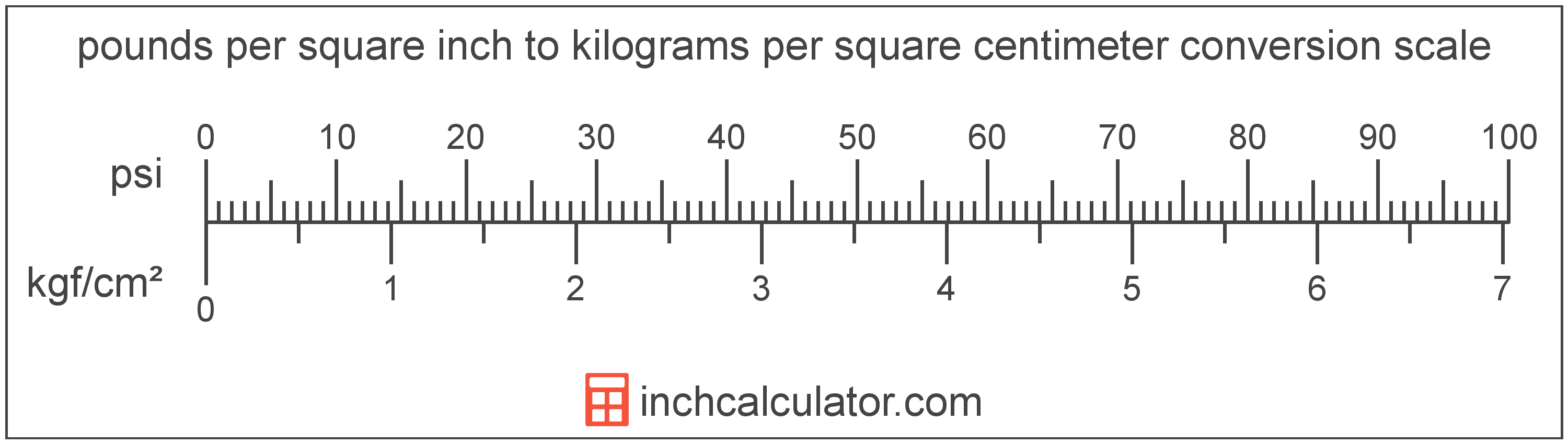# Kilograms per Square Centimeter to Pounds per Square Inch Converter

Enter the pressure in kilograms per square centimeter below to get the value converted to pounds per square inch.

Results in Pounds per Square Inch:1 kgf/cm² = 14.223358 psi

## How to Convert Kilograms per Square Centimeter to Pounds per Square Inch

To convert a measurement in kilograms per square centimeter to a measurement in pounds per square inch, multiply the pressure by the following conversion ratio: 14.223358 pounds per square inch/kilogram per square centimeter.

Since one kilogram per square centimeter is equal to 14.223358 pounds per square inch, you can use this simple formula to convert:

pounds per square inch = kilograms per square centimeter × 14.223358

The pressure in pounds per square inch is equal to the pressure in kilograms per square centimeter multiplied by 14.223358.

For example, here's how to convert 5 kilograms per square centimeter to pounds per square inch using the formula above.
pounds per square inch = (5 kgf/cm² × 14.223358) = 71.116792 psiKilograms per square centimeter and pounds per square inch are both units used to measure pressure. Keep reading to learn more about each unit of measure.

## What Are Kilograms per Square Centimeter?

One kilogram per square centimeter is the pressure of equal to one kilogram-force per square centimeter.

The kilogram per square centimeter is a non-SI metric unit for pressure. A kilogram per square centimeter is sometimes also referred to as a kilogram per square centimetre or kilogram-force per centimeter square. Kilograms per square centimeter can be abbreviated as kgf/cm²; for example, 1 kilogram per square centimeter can be written as 1 kgf/cm².

In the expressions of units, the slash, or solidus (/), is used to express a change in one or more units relative to a change in one or more other units. For example, kgf/cm² is expressing a change in weight relative to a change in area.

The unit is deprecated and not permitted for use with SI units, although it is still in use as some precision measuring devices still use the unit.

Kilograms per square centimeter can be expressed using the formula:
1 kgf/cm2 = 1 kgf / cm2

Pressure in kilograms per square centimeter are equal to the kilogram-force divided by the area in square centimeters.

## What Are Pounds per Square Inch?

One pound per square inch is the pressure of equal to one pound-force per square inch.

The pound per square inch is a US customary and imperial unit of pressure. A pound per square inch is sometimes also referred to as a pound-force per square inch. Pounds per square inch can be abbreviated as psi; for example, 1 pound per square inch can be written as 1 psi.

PSI can be expressed using the formula:
1 psi = 1 lbf / in2

Pressure in pounds per square inch are equal to the pound-force divided by the area in square inches.

## Kilogram per Square Centimeter to Pound per Square Inch Conversion Table

Table showing various kilogram per square centimeter measurements converted to pounds per square inch.
Kilograms Per Square Centimeter Pounds Per Square Inch
1 kgf/cm² 14.22 psi
2 kgf/cm² 28.45 psi
3 kgf/cm² 42.67 psi
4 kgf/cm² 56.89 psi
5 kgf/cm² 71.12 psi
6 kgf/cm² 85.34 psi
7 kgf/cm² 99.56 psi
8 kgf/cm² 113.79 psi
9 kgf/cm² 128.01 psi
10 kgf/cm² 142.23 psi
11 kgf/cm² 156.46 psi
12 kgf/cm² 170.68 psi
13 kgf/cm² 184.9 psi
14 kgf/cm² 199.13 psi
15 kgf/cm² 213.35 psi
16 kgf/cm² 227.57 psi
17 kgf/cm² 241.8 psi
18 kgf/cm² 256.02 psi
19 kgf/cm² 270.24 psi
20 kgf/cm² 284.47 psi
21 kgf/cm² 298.69 psi
22 kgf/cm² 312.91 psi
23 kgf/cm² 327.14 psi
24 kgf/cm² 341.36 psi
25 kgf/cm² 355.58 psi
26 kgf/cm² 369.81 psi
27 kgf/cm² 384.03 psi
28 kgf/cm² 398.25 psi
29 kgf/cm² 412.48 psi
30 kgf/cm² 426.7 psi
31 kgf/cm² 440.92 psi
32 kgf/cm² 455.15 psi
33 kgf/cm² 469.37 psi
34 kgf/cm² 483.59 psi
35 kgf/cm² 497.82 psi
36 kgf/cm² 512.04 psi
37 kgf/cm² 526.26 psi
38 kgf/cm² 540.49 psi
39 kgf/cm² 554.71 psi
40 kgf/cm² 568.93 psi

## References

1. National Institute of Standards and Technology, NIST Guide to the SI, Chapter 6: Rules and Style Conventions for Printing and Using Units, https://www.nist.gov/pml/special-publication-811/nist-guide-si-chapter-6-rules-and-style-conventions-printing-and-using